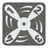Repeating Decimals
Elementary+
English

In mathematics, a repeating decimal is a way of representing a rational number. A decimal representation of a number is called a repeating decimal if at some point there is some finite sequence of digits that is repeated infinitely. For example: the decimal representation of 1/3 = 0.3333333… or 0.(3) becomes periodic just after the decimal point, repeating the single-digit sequence "3" infinitely. Here is another example is 27/26 = 1.0384615384615385… or 1.0(384615), where the decimal becomes periodic after the second digit past the decimal point, repeating the sequence "384615" infinitely. Rational numbers are numbers which can be expressed in the form a/b where a and b are integers and b is not zero. This form is known as a common fraction. Read more about this at Wikipedia .

You should write a function for the converting a fraction into decimal representation. If the decimal is repeating, you should represent it using the brackets format seen above. You are given two integers, the first is the fractions numerator and the second is its denominator. For this task, you will need to return the fraction in decimal representation as a string. The integer results (as 0 or 2) must be ended with a dot.

Fraction Ellipsis Brackets
1/3 0.333333... 0.(3)
5/3 1.666666... 1.(6)
3/8 0.375 0.375
7/11 0.636363... 0.(63)
29/12 2.41666666... 2.41(6)
11/7 1.571428571428... 1.(571428)

Input: Two arguments. A numerator and a denominator as integers.

Output: The decimal representation of the fraction in the bracket format as a string.

Example:

```convert(1, 3) == "0.(3)"
convert(5, 3) == "1.(6)"
convert(3, 8) == "0.375"
convert(7, 11) == "0.(63)"
convert(29, 12) == "2.41(6)"
convert(11, 7) == "1.(571428)"
convert(0, 117) == "0."
convert(4, 2) == "2."
```

How it is used: This is the important part for mathematical software. And of you need to help your children with homework.

Precondition:
0 ≤ numerator ≤ 1000
1 ≤ denominator ≤ 1000

## Become Awesome

• No Limits
• More Content45
Settings
Code:
Other:
CheckiO Extensions

CheckiO Extensions allow you to use local files to solve missions. More info in a blog post.

In order to install CheckiO client you'll need installed Python (version at least 3.8)

Install CheckiO Client first:

`pip3 install checkio_client`

`checkio --domain=py config --key=`

Sync solutions into your local folder

`checkio sync`

(in beta testing) Launch local server so your browser can use it and sync solution between local file end extension on the fly. (doesn't work for safari)

`checkio serv -d`

Alternatevly, you can install Chrome extension or FF addon

`checkio install-plugin`
`checkio install-plugin --ff`
`checkio install-plugin --chromium`

Read more here about other functionality that the checkio client provides. Feel free to submit an issue in case of any difficulties.